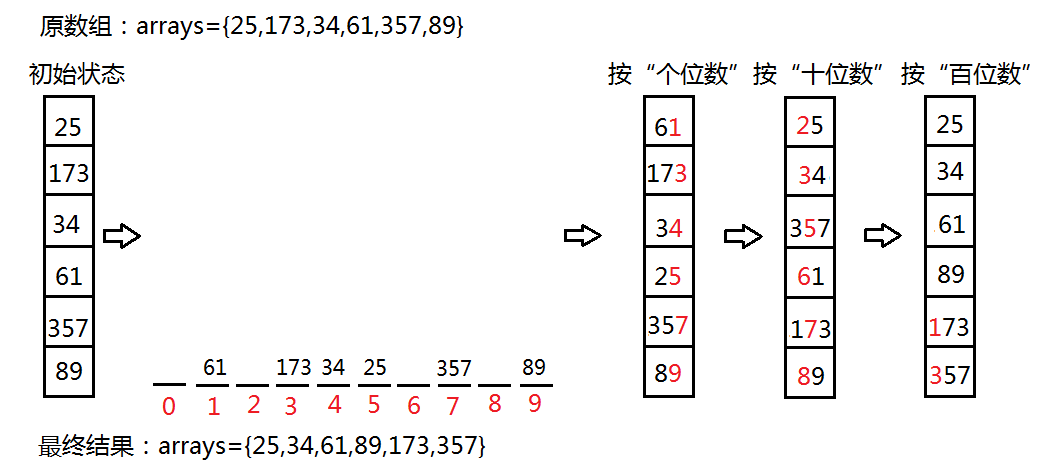# Java八大排序之基数排序

一，求出一个数组中最大的数的位数，表示要排序多少次；

二，先根据个位数排序，个位数相同的放入相同的桶子中，分别对应着0-9的桶子；

三，然后将桶子中的数据重新串连起来，按照0-9的桶子顺序和先进先出的规则；

四，重复第二步和第三步，只是之后的个位数分别设为十位数，百位数，千位数......，直到排序次数完结。1 public class RadixSort {
2     //最大数的位数
3     public static int maxLength(int[] arrays){
4         int maxArr = arrays;
5         for (int i = 0; i < arrays.length-1; i++){
6             if (arrays[i] < arrays[i+1]){
7                 maxArr = arrays[i+1];
8             }
9         }
10         return (maxArr+"").length();
11     }
12     //排序
13     public static void radix(int[] arrays){
14         int i = 0;
15         int j;
16         int n = 1;
17         int index = 0;  //数组下标
18         int l = arrays.length;  //数组长度
19         int max = maxLength(arrays);    //最大数的位数
20         //创建十个桶子数组
21         int[][] tempArr = new int[l];
22         //每个桶子的定义
23         int[] counts = new int;
24         while (i <= max){
25             //把arrays数组中的数据分配到每个数组中
26             for (j = 0; j < l; j++){
27                 int temp = arrays[j]/n%10;
28                 tempArr[temp][counts[temp]] = arrays[j];
29                 counts[temp]++;
30             }
31             //将每个桶子数组中的数据收集到arrays数组中
32             for (j = 0; j < counts.length; j++){
33                 for (int a = 0; a < counts[j]; a++){
34                     arrays[index] = tempArr[j][a];
35                     index++;
36                 }
37                 counts[j] = 0;
38             }
39             index = 0;
40             n = n*10;
41             i++;
42         }
43     }
44     //测试
45     public static void main(String[] args) {
46         int[] arrays={49,38,65,97,176,213,227,49,78,34,12,164,11,18,1};
50 }
[1, 11, 12, 18, 34, 38, 49, 49, 65, 78, 97, 164, 176, 213, 227]